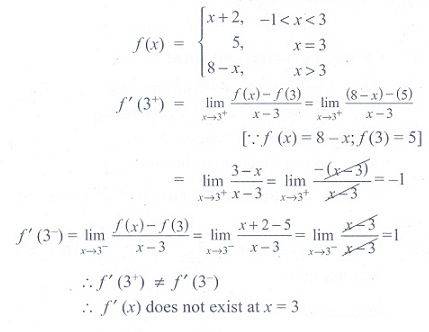# Chapter 10: Differential Calculus Differentiability and Methods of Differentiation - Online Test

Q1. If x = asinθ and y = bcosθ , then d2y/ d2x is
Answer : Option C
Explaination / Solution: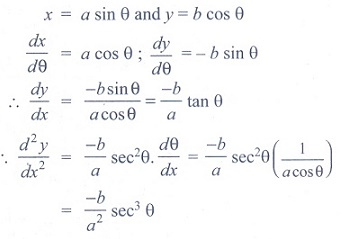Q2. The differential coefficient of log10x with respect to logx10 is
Answer : Option B
Explaination / Solution: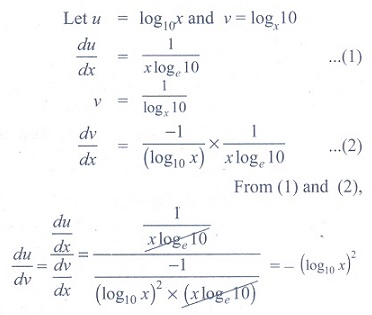Q3. If f (x) = x + 2, then f '( f (x)) at x = 4 is
Answer : Option B
Explaination / Solution: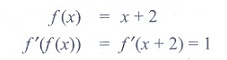Q4. If y = (1− x)2/ x2 , then dy/ dx is
Answer : Option D
Explaination / Solution: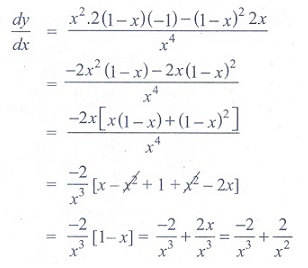Q5. If pv = 81, then dp/dv at v = 9 is
Answer : Option B
Explaination / Solution: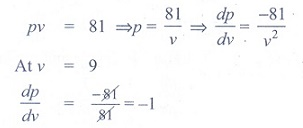Q6. If  f (x ) =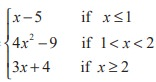, then the right hand derivative of f(x) at x = 2 is
Answer : Option C
Explaination / Solution: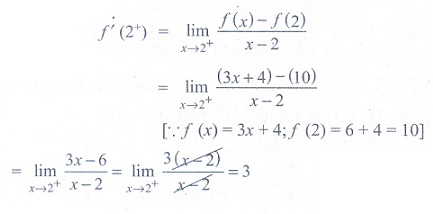Q7. It is given that f '(a) exists, then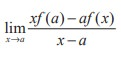is
Answer : Option A
Explaination / Solution: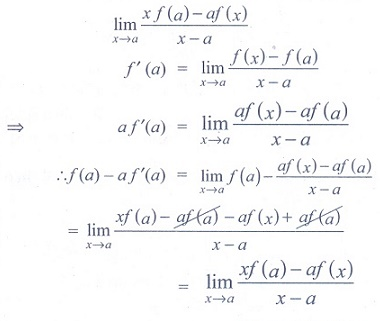Q8. If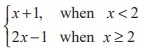, then f '(2) is
Answer : Option D
Explaination / Solution: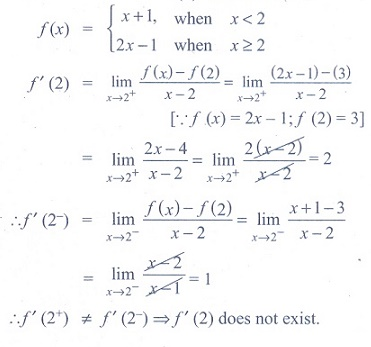Q9. If g (x) = (x2 + 2x + 3) f (x) and f (0) = 5 and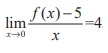, then g '(0) is
Answer : Option B
Explaination / Solution:
No Explaination.

Q10. If f(x) =, then at x = 3, f '(x) is
Answer : Option D
Explaination / Solution: